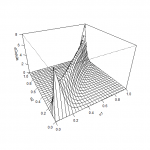Want to share your content on R-bloggers? click here if you have a blog, or here if you don't.Copulas are a powerful statistical tool commonly used in the finance sector to generate samples from a given multivariate joint distribution.
The principal advantage of using those types of function over other methods is that copulas describe the multivariate joint distribution as his margin and the dependence structure between them,
which give the user the power to fine tune his model component by component.
For example, if you have two independent variables of known distribution `X_1` and `X_2` which interact to create a dependant
variable `Y` you can set `X_1` and `X_2` as the margin of the distribution of `Y` and find the appropriate copula to simulate the interaction between the margins and fit the data.

In the previous post we’ve seen how to create a copula object and how to generate sample with the most commonly used copula.
In this post we’ll learn how to use choose a copula that fit your data and how to make a rough estimate of the probability of a given event.

To be able to do those exercises, you must have installed the packages `ggplot2`,`fitdistrplus`, `VineCopula` and `copula`. Also, you can find the dataset we’ll use
for this set of exercises here. It’s a clean dataset of the daily return of the Apple and Microsoft from
May 2000 to May 2017.

Answers to the exercises are available here.

Exercise 1
We’ll start by fitting the margin. First, do a histogram of both Apple and Microsoft returns to see the shape of both distributions.

Exercise 2
Both distributions seems symmetric and have a domain which contain positive and negative values.
Knowing those facts, use the `fitdist()` function to see how the normal, logistic and Cauchy distribution fit the Apple returns dataset.
Which of those three distributions is best suited to simulate the Apple return dataset and what are the parameter of this distribution?

Exercise 3
Repeat exercise 2 with the Microsoft return.

Exercise 4
Plot the joint distribution of the Apple and Microsoft daily returns. Add the regression line to the plot and compute the correlation of both variables.

Exercise 5
Use the `pobs()` from the `VineCopula()` package to compute the pseudo-observations for both returns values, then use the `BiCopSelect()` function to select the copula
which minimise the AIC on the dataset. Which copula is selected and what are his parameters.

Learn more about MultiVariate analysis in the online course Case Studies in Data Mining with R. In this course you will work thru a case study related to multivariate analysis and how to work with forecasting in the S&P 500.

Exercise 6
Use the appropriate function from the `VineCopula()` package to create a copula object with the parameter computed in the last exercise. Then, do a three dimensional plot and a contour plot of the copula.

Exercise 7
Set the seed to 42 and generate a sample of 1000 points from this copula. Plot the sample and calculate the correlation of this sample.
Does the correlation of the sample is similar to the correlation between the Apple and Microsoft returns?

Exercise 8
Create a distribution from the copula you selected and the margins you fitted in the exercise 2 and 3.

Exercise 9
Generate 1000 points from the distribution of exercise 8 and plot those points, with the Apple and Microsoft returns, in the same plot.

Exercise 10
Having made a model, let’s make some crude estimation with it! Imagine that this model has been proven to be effective to describe the relation between the apple return and
the Microsoft return for a considerable amound of time and there’s a spike in the price of Apple stock. Suppose you have another model who describe the Apple stock and who
lead you to believe that the daily return on this stock has a 90% chance to be between 0.038 and 0.045. Using only this information, compute the range containing the possible
daily return of the Microsoft stock at the end of the day and the mean of the possible values.